# 新品发布 | 交流微网一体式电控装置——50kW*N级交流电能路由器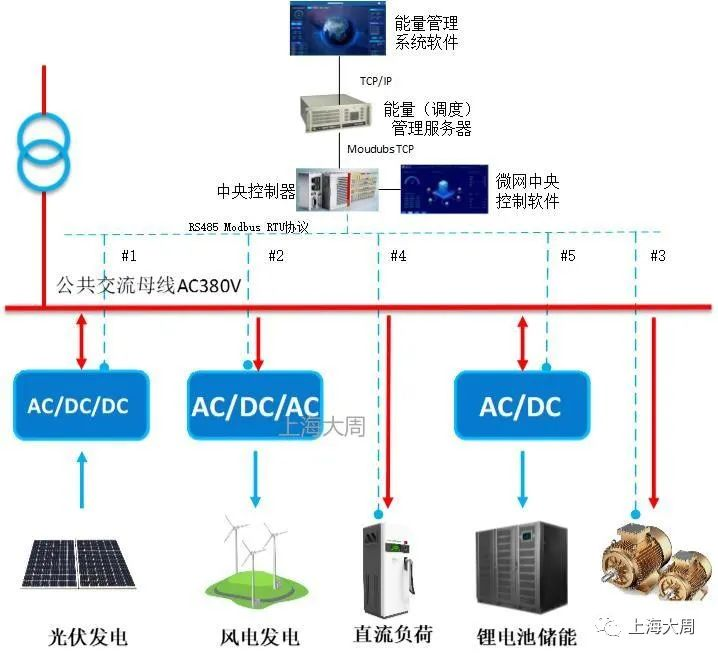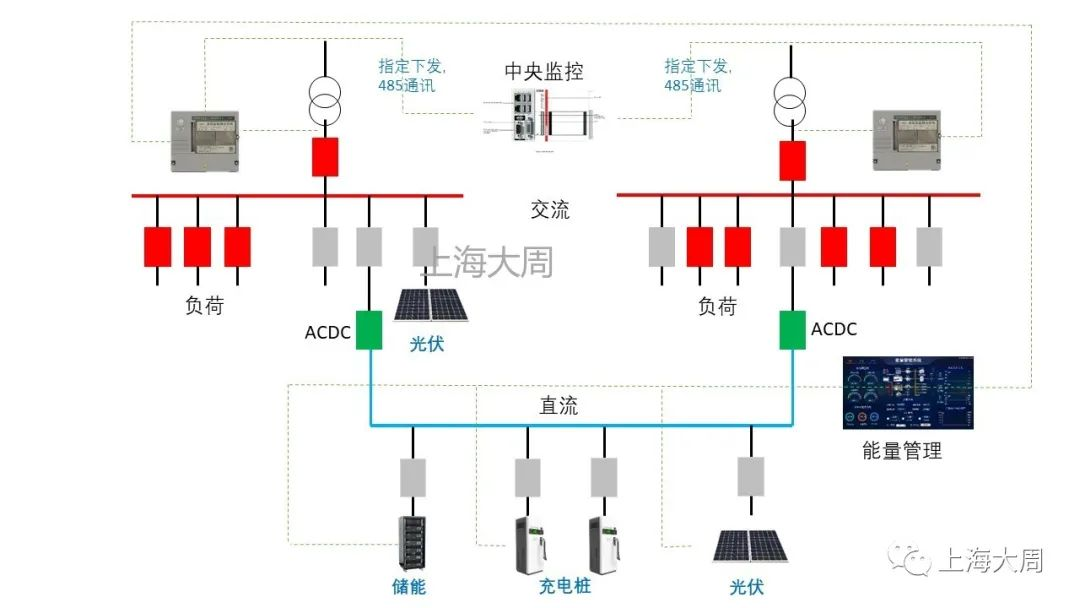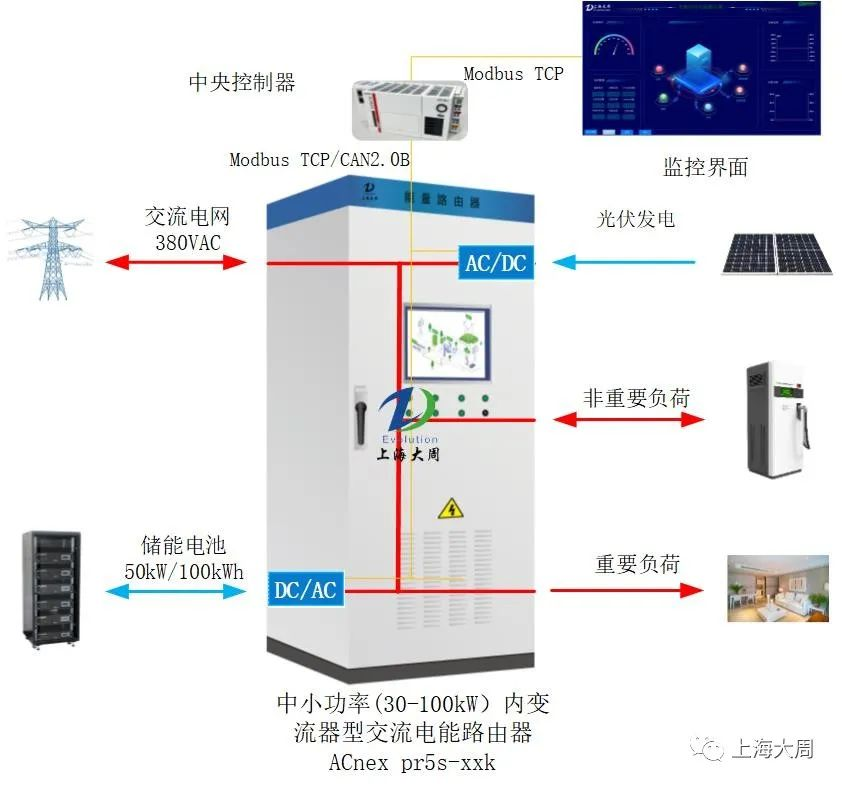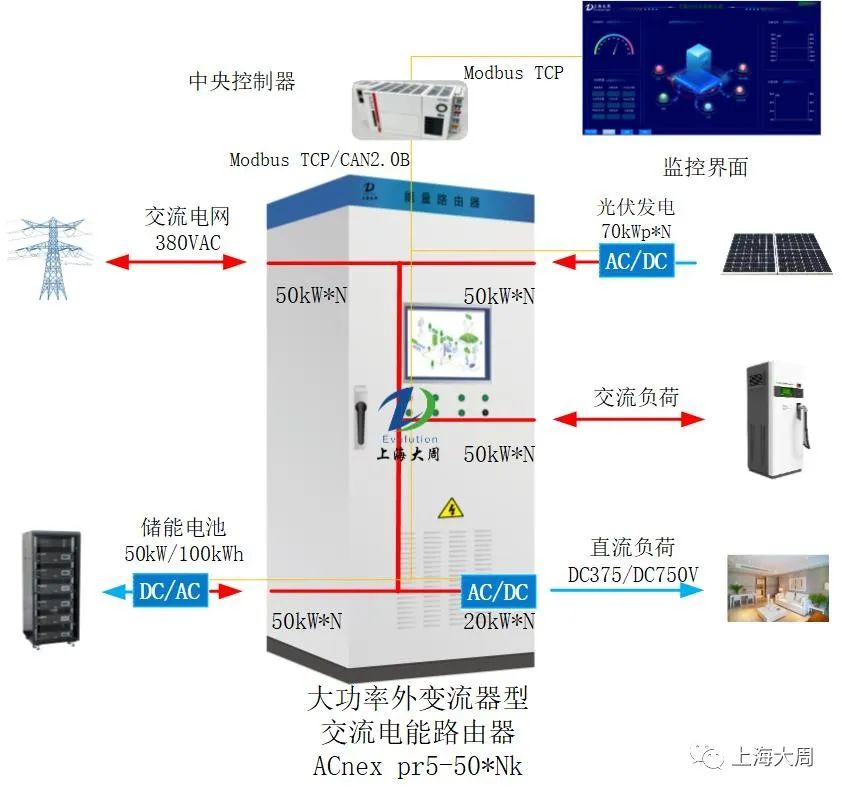01交流微电网<<<<

02电能路由器<<<<

03市场化电源<<<<

04可升级能力<<<<

05 可应急供电<<<<

1

2

3

4

5

6

7

8

9

10

11

（另选）云端能量管理系统，非标配

(1) 多能互补并离网智能控制

(2) 在线EPS功能控制

(3) 离网交流母线稳定控制

(4) 系统功率平衡控制

(5) 系统云能量管理（另选）

(6) 电池充放电时段设置

(7) 与上层管理系统通讯

(8) 智能充放电与电池保护

(9) 电量统计

(10) 数据检测

(11) 负荷控制

(12) 人机交互监控

(13) 用户权限管理

(14) 运行数据显示与存储

(15) 故障报警处理

(16) 操作记录查询

(17) 二次开发

01在网模式<<<<

02独立模式<<<<

03光伏发电<<<<

04削峰填谷<<<<

05虚拟电厂<<<<

06支持黑启动<<<<

07模式切换<<<<

08UPS功能<<<

09电能质量补偿<<<<

10与上层管理系统通讯<<<<

11 电网调度指令响应控制（选配）<<<<

12数据接口开放<<<<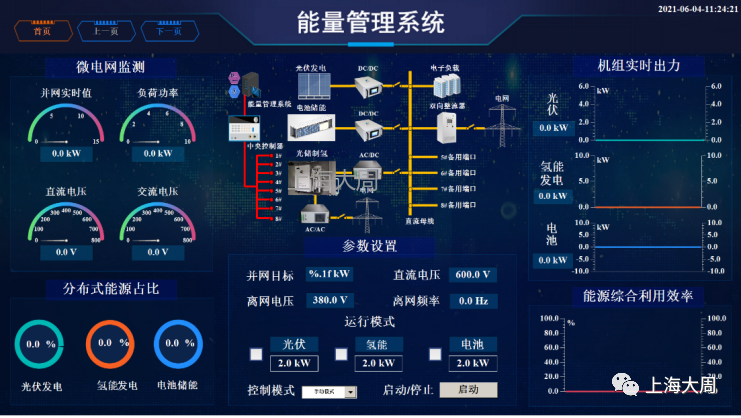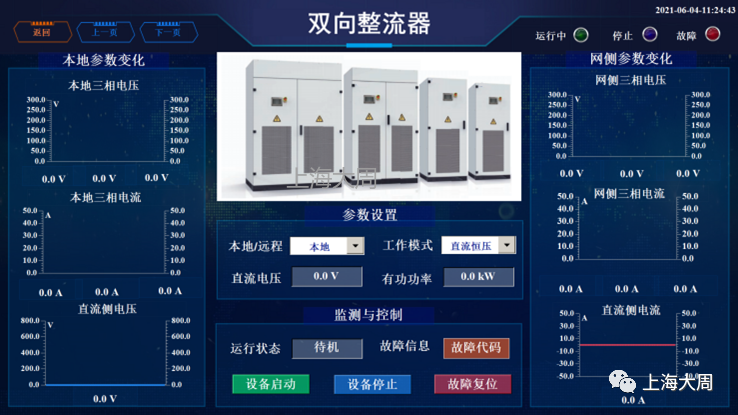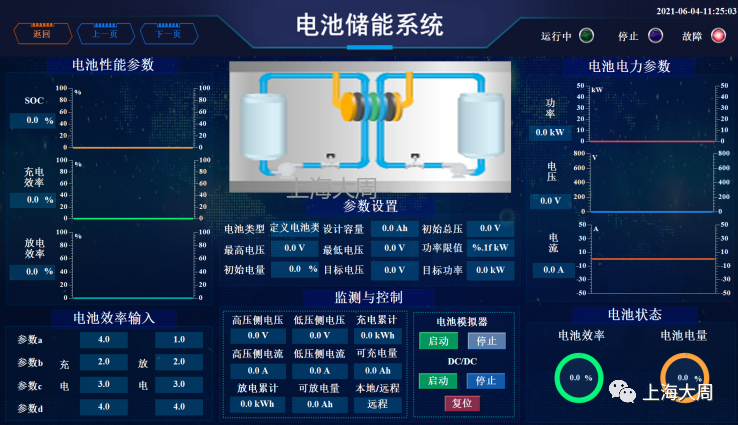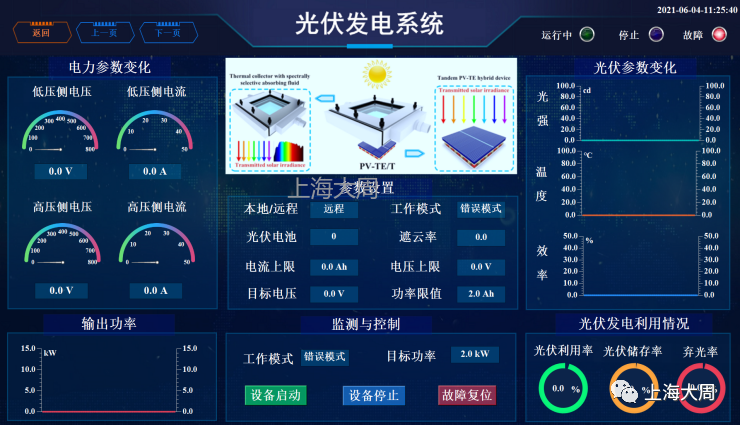工作时间

联系方式